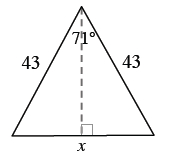### Home > INT2 > Chapter 7 > Lesson 7.2.2 > Problem7-94

7-94.

For each polygon below, use the geometric relationships to solve for the given variable(s). Show all work. Name the geometric relationships you used.

1.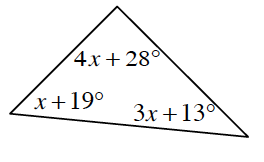$4x + 28^\circ + x + 19^\circ + 3x + 13^\circ = 180^\circ$

$x = 15^\circ$ (Be sure to name the geometric relationship(s) you used.

1.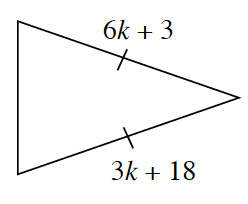$6k + 3 = 3k + 18$

1.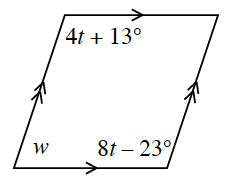This is a parallelogram. What do you know about the opposite angles of a parallogram?

$w = 131^\circ$

1.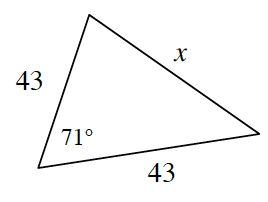Use the tangent ratio to write an equation and solve for half of $x$.